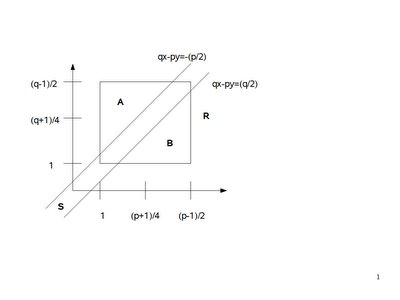## Wednesday, February 15, 2006

In today's blog I continue the proof for the Principle of Quadratic Reciprocity. If you are not familiar with the concepts of quadratic reciprocity, quadratic residues, or the Legendre Symbol start here.

If you would like to start this proof at the beginning, start here.

Today's proof is taken from Gareth A. Jones and J. Mary Jones' Elementary Number Theory.

Lemma 1:
(a) Let x' = (p+1)/2 - x
(b) Let y' = (q+1)/2 - y
Then
qx - py is greater than -(p/2) if and only if qx' - py' is less than q/2.

(1) Assume that qx - py is greater than (-p/2)

(2) (p/2) + qx - py is greater than 0

(3) (p +q-q+pq-pq)/2 + qx - py is greater than 0

(4) (p - q + pq - pq)/2 + qx - py is greater than (-q/2)

(5) -q(p+1)/2 + p(q+1)/2 + qx - py is greater than (-q/2)

(6) q(p+1)/2 - p(q+1)/2 - qx + py is less than (q/2)

(7) q[(p+1)/2 - x] - p[(q+1)/2 - y] is less than (q/2)

(8) qx' - py' is less than (q/2)

(9) We can use the same reasoning to show that:
if qx' - py is less than (q/2), then qx - py is greater than (-p)/2.

QED

Lemma 2: Given:
(a) Let P = { 1, 2, ... (p-1)/2 }
(b) Let Q = {1, 2, ... (q-1)/2 }
(c) Let A be the set of points PxQ such that qx-py is greater than (-p)/2. [See diagram in Lemma 3]
(d) Let B be the set of points PxQ such that qx-py is less than (q/2) [See diagram in Lemma 3]
(e) Let α be the number of points in A
(f) Let β be the number of points in B
Then:
There is a one-to-one mapping between α and β [So that, they both have the same number of points]

Proof:

(1) Let's define the following function:
p(x,y) = (x',y') = [ (p+1)/2 - x, (q+1)/2 - y ]

(2) p(A) = p(B) since qx - py is greater than (-p)/2 implies that qx' - py is less than (q/2). [See Lemma 1 above]

(3) p(B) = p(A) since qx - py is less than (q/2) implies that qx' - py' is greater than (-p)/2. [See Lemma 1 above]

QED

Lemma 3: Given
(a) p,q are distinct, odd primes
(b) P is a set = { 1, 2, ... (p-1)/2 }
(c) Q is a set = { 1, 2, ... (q-1)/2 }
(d) x,y are integers such that x ∈ P and y ∈ Q
(e) (-p/2) is less than qx - py which is less than (q/2).
(f) μ = the number of elements (x,y) ∈ P x Q where (-p/2) is less than qx - py which is less than -1.
(g) ν = the number of elements (y,x) ∈ Q x P where (-q/2) is less than py - qx which is less than -1.

Then:
(-1)μ + ν = (-1)[(p-1)(q-1)]/4

Proof:

(1) The set of assumptions can be represented as a graph:We see that:
(a) R is the set of all points PxQ = [(p-1)/2][(q-1)/2] = (p-1)(q-1)/4

(b) μ + ν = R ∩ S

(c) A = the area of PxQ that falls above S

(d) B = the area of PxQ that falls below S

(2) We can further define α, β such that:

α = the number of points ∈ A
β = the number of points ∈ B

(3) From this, we see that the number of points R ∩ S is:
(p-1)(q-1)/4 - (α + β)

(4) Now we can show that α = β or in other words that there is a one-to-one mapping between elements in α with elements in β by the following:

(a) We can do this using the following rotation p(x,y):

p(x,y) = (x',y') = ( [p+1]/2 - x, [q+1]/2 - y )

(b) So, for example, the corners of R before the rotation are: (1,[q-1]/2), ([p-1]/2,[q-1]/2), (1,1), and ([p-1]/2, 1).

(c) After the rotation, the corners of R' are: ([p-1]/2,1), (1,1), ([p-1]/2,[q-1]/2), and (1,[q-1]/2).

(d) So, we see that R' has the same corners as R.

(e) By Lemma 2, we see that there is a one-to-one correspondence between A and B.

(5) So, this means that α + β ≡ 2*α ≡ 0 (mod 2).

(6) But this means that the α + β does not change the result since:
(-1)μ + ν = (-1)μ + ν*(-1)

So:
(-1)μ + ν = (-1)μ + ν + 2*α = (-1)(p-1)(q-1)/4

QED